# Force Exerted by a Uniform-Density Shell or Sphere

## Tohline 1982

### General Derivation from Notes Dated 29 November 1982

If the force per unit mass exerted at the position,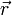$~\vec{r}$, from a single point mass,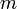$~m$, is given by,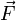$~\vec{F}$$~=$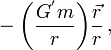$~- \biggl( \frac{G^'m}{r} \biggr) \frac{\vec{r}}{r} \, ,$

then the force per unit mass exerted at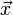$~\vec{x}$ by a continuous mass distribution, whose mass density is defined by the function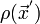$~\rho(\vec{x}^')$, is,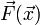$~\vec{F}(\vec{x})$$~=$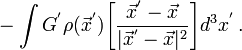$~- \int G^' \rho(\vec{x}^') \biggl[ \frac{\vec{x}^' - \vec{x}}{| \vec{x}^' - \vec{x} |^2} \biggr] d^3x^' \, .$

This central force can also be expressed in terms of the gradient of a scalar potential,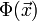$~\Phi(\vec{x})$, specifically,$~\vec{F}(\vec{x})$$~=$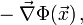$~- \vec\nabla\Phi(\vec{x}) \, ,$

where,$~\Phi(\vec{x})$$~=$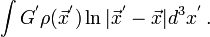$~ \int G^' \rho(\vec{x}^') \ln | \vec{x}^' - \vec{x} | d^3x^' \, .$

For a spherically symmetric mass distribution,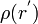$~\rho(r^')$, the magnitude of the force that is directed along the radial vector,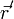$~\vec{r}^'$, and measured from the center of the mass distribution can be expressed as the following single integral over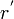$~r^'$: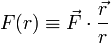$~F(r) \equiv \vec{F}\cdot \frac{\vec{r}}{r}$$~=$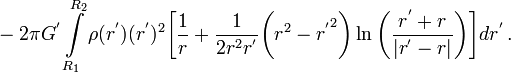$~ -2\pi G^' \int\limits_{R_1}^{R_2} \rho(r^') (r^')^2 \biggl[\frac{1}{r} + \frac{1}{2r^2 r^'} \biggl( r^2 - {r^'}^2 \biggr) \ln\biggl( \frac{r^' + r}{|r^' - r|} \biggr) \biggr] dr^' \, .$

This integral can be completed analytically if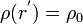$~\rho(r^') = \rho_0$, that is, for a uniform-density mass distribution. Independent of whether the limits of integration,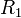$~R_1$ and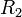$~R_2$, are less than or greater than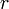$~r$, the integral gives,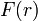$~F(r)$$~=$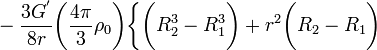$~ - \frac{3G^'}{8r} \biggl( \frac{4\pi}{3}\rho_0 \biggr) \biggl\{ \biggl( R_2^3 - R_1^3 \biggr) + r^2 \biggl(R_2 - R_1\biggr)$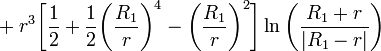$~ + r^3 \biggl[ \frac{1}{2} + \frac{1}{2}\biggl( \frac{R_1}{r} \biggr)^4 - \biggl( \frac{R_1}{r} \biggr)^2\biggr] \ln\biggl( \frac{R_1 + r}{|R_1 - r|} \biggr)$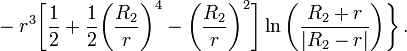$~ - r^3 \biggl[ \frac{1}{2} + \frac{1}{2}\biggl( \frac{R_2}{r} \biggr)^4 - \biggl( \frac{R_2}{r} \biggr)^2\biggr] \ln\biggl( \frac{R_2 + r}{|R_2 - r|} \biggr) \biggr\} \, .$

If the position,$~r$, is located outside of a uniform-density sphere, then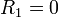$~R_1 = 0$ and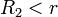$~R_2 < r$, so the aggregate acceleration becomes,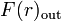$~F(r)_\mathrm{out}$$~=$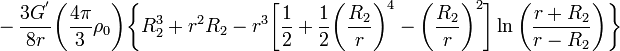$~ - \frac{3G^'}{8r} \biggl( \frac{4\pi}{3}\rho_0 \biggr) \biggl\{ R_2^3 + r^2 R_2 - r^3 \biggl[ \frac{1}{2} + \frac{1}{2}\biggl( \frac{R_2}{r} \biggr)^4 - \biggl( \frac{R_2}{r} \biggr)^2\biggr] \ln\biggl( \frac{r+R_2}{r- R_2} \biggr) \biggr\}$$~=$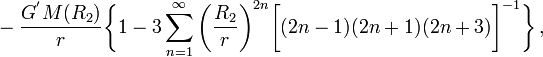$~ - \frac{G^' M(R_2)}{r} \biggl\{ 1 - 3 \sum_{n=1}^{\infty} \biggl( \frac{R_2}{r} \biggr)^{2n} \biggl[(2n-1)(2n+1)(2n+3) \biggr]^{-1} \biggr\} \, ,$

where,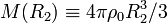$~M(R_2) \equiv 4\pi \rho_0 R_2^3/3$. If the position,$~r$, is located interior to a uniform-density shell, then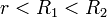$~r < R_1 < R_2$ and the aggregate acceleration is,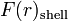$~F(r)_\mathrm{shell}$$~=$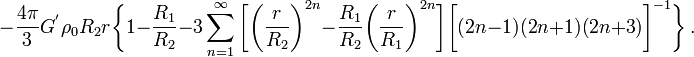$~ - \frac{4\pi}{3} G^' \rho_0 R_2 r \biggl\{1 - \frac{R_1}{R_2} - 3 \sum_{n=1}^{\infty} \biggl[ \biggl( \frac{r}{R_2} \biggr)^{2n} - \frac{R_1}{R_2} \biggl( \frac{r}{R_1} \biggr)^{2n}\biggr] \biggl[(2n-1)(2n+1)(2n+3) \biggr]^{-1} \biggr\} \, .$

If$~r$ is inside a uniform-density sphere, then$~R_1 = 0$ and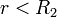$~ r < R_2$, so the aggregate acceleration is,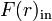$~F(r)_\mathrm{in}$$~=$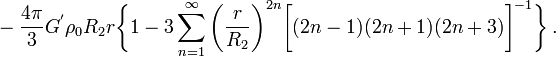$~ - \frac{4\pi}{3} G^' \rho_0 R_2 r \biggl\{ 1 - 3 \sum_{n=1}^{\infty} \biggl( \frac{r}{R_2} \biggr)^{2n} \biggl[(2n-1)(2n+1)(2n+3) \biggr]^{-1} \biggr\} \, .$

### Limiting Cases

Some limiting cases are of interest for the uniform sphere, i.e., when$~R_1 = 0$. First, notice that (Gradshteyn & Ryzhik 1965, formula 0.141-2),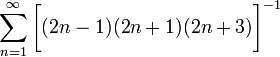$~\sum_{n=1}^{\infty} \biggl[(2n-1)(2n+1)(2n+3) \biggr]^{-1}$$~=$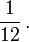$~ \frac{1}{12} \, .$

Sitting on the Surface: Therefore, when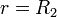$~r = R_2$ — that is, on the surface of the uniform-density sphere,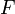$~F$$~=$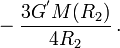$~ - \frac{3 G^' M(R_2)}{4R_2} \, .$

So the force acts as though the mass is all concentrated at a point, not at the center of the sphere, but at a distance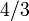$~4/3$ of the sphere's radius away.

Well Inside the Surface: When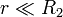$~r \ll R_2$,$~F(r)_\mathrm{in}$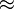$~\approx$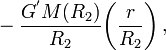$~ - \frac{G^' M(R_2)}{R_2} \biggl( \frac{r}{R_2} \biggr) \, ,$

that is, the acceleration grows linearly with$~r$, as in any harmonic potential.

Well Outside the Sphere: When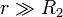$~r \gg R_2$,$~F(r)_\mathrm{out}$$~\approx$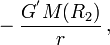$~ - \frac{G^' M(R_2)}{r} \, ,$

which is in line with the adopted point-mass specification.